# Intermediate Algebra Tutorial 12

Intermediate Algebra
Tutorial 12: Graphing EquationsAnswer/Discussion to 1a
A(3, 1), B(-2, -1/2), C(2, -2), and D(0,1)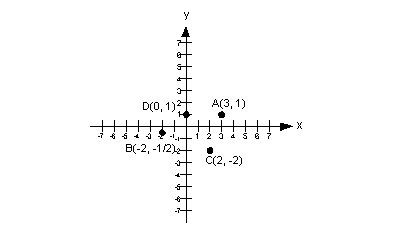A(3, 1)  lies in quadrant I.

B(-2, -1/2)  lies in quadrant III.

C(2, -2)  lies in quadrant IV.

D(0, 1)  lies on the y axis.Answer/Discussion to 2a
y = 4x - 10     (0, -10), (1, -14), (-1, -14)

Which number is the x value and which one is the y value?  If you said x = 0 and y = -10, you are correct!

Let's plug (0, -10) into the equation and see what we get: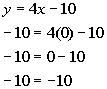*Plug in 0 for x and -10 for y

This is a TRUE statement, so (0, -10) is a solution to the equation y = 4x - 10.

Now, let's take a look at (1, -14).

Which number is the x value and which one is the y value?    If you said x = 1 and y = -14, you are right!

Let's plug (1, -14) into the equation and see what we get: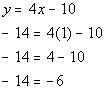*Plug in 1 for x and -14 for y

This is a FALSE statement, so (1, -14) is NOT a solution to the equation y = 4x - 10.

Now, let's take a look at (-1, -14).

Which number is the x value and which one is the y value?    If you said x = -1 and y = -14, you are right!

Let's plug (-1, -14) into the equation and see what we get: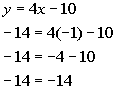*Plug in -1 for x and -14 for y

This is a TRUE statement, so (-1, -14) is  a solution to the equation y = 4x - 10.Answer/Discussion to 3a
y = 2x - 1

If we subtract 2x from both sides, then we can write the given equation as -2x + y = -1.

Since we can write it in the standard form, Ax + By = C, then we have a linear equation.

This means that we will have a line when we go to graph this.

The three x values I'm going to use are -1, 0, and 1.  (Note that you can pick ANY three x values that you want.  You do not have to use the values that I picked.) You want to keep it as simple as possible.  The following is the chart I ended up with after plugging in the values I mentioned for x.

x y = 2x - 1 (x, y) -1 y = 2(-1) - 1 = -3 (-1, -3) 0 y = 2(0) - 1 = -1 (0, -1) 1 y = 2(1) - 1 = 1 (1, 1)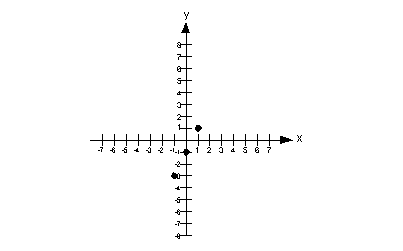Step 3:  Draw the graph.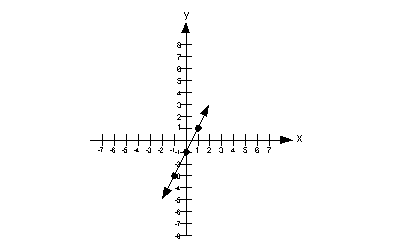Answer/Discussion to 3b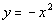If we add  x squared to both sides we would end up with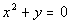.   Is this a linear equation?  Note how we have an x squared as opposed to x to the one power.

It looks like we cannot write it in the form Ax + By = C, because the x has to be to the one power, not squared.  So this is not a linear equation.

However, we can still graph it.

The seven x values that I'm going to use are -3, -2, -1, 0, 1, 2, and 3.  (Note that you can pick ANY x values that you want.  You do not have to use the values that I picked.) You want to keep it as simple as possible.  The following is the chart I ended up with after plugging in the values I mentioned for x.

x(x, y) -3 y = -(-3)^2 = -9 (-3, -9) -2 y = -(-2)^2  = -4 (-2, -4) -1 y = -(-1)^2 = -1 (-1, -1) 0 y = -(0)^2 = 0 (0, 0) 1 y = -(1)^2 = -1 (1, -1) 2 y = -(2)^2 = -4  (2, -4) 3 y = -(3)^2 = -9 (3, -9)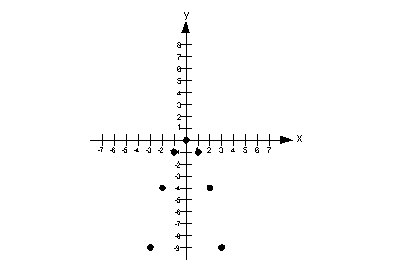Step 3:  Draw the graph.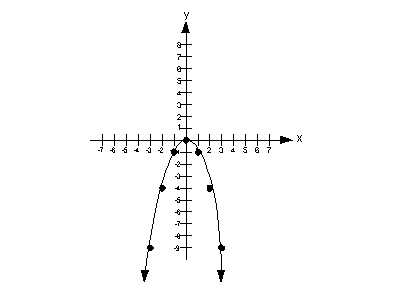Answer/Discussion to 3c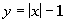Do you think this equation is linear or not?  It is a tricky problem, because both the x and y variables are to the one power.  However, x is inside the absolute value sign and we can't just take it out of there.

In other words, we can't write it in the form Ax + By = C.  This means that this equation is not a linear equation.

The seven x values that I'm going to use are -3, -2, -1, 0, 1, 2, and 3.  (Note that you can pick ANY x values that you want.  You do not have to use the values that I picked.) You want to keep it as simple as possible.  The following is the chart I ended up with after plugging in the values I mentioned for x.

x(x, y) -3 y = |-3| - 1 = 2 (-3, 2) -2 y = |-2| - 1 = 1 (-2, 1) -1 y = |-1| - 1 = 0 (-1, 0) 0 y = |0| - 1 = -1 (0, -1) 1 y = |1| - 1 = 0 (1, 0) 2 y = |2| - 1 = 1 (2, 1) 3 y = |3| - 1 = 2 (3, 2)Step 3:  Draw the graph.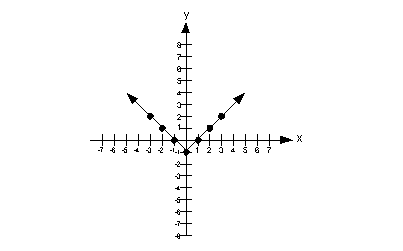Last revised on July 3, 2011 by Kim Seward.